# Bihar Board Class 12 Physics Solutions Chapter 12 Atoms

Bihar Board Class 12 Physics Solutions Chapter 12 Atoms Textbook Questions and Answers, Additional Important Questions, Notes.

## BSEB Bihar Board Class 12 Physics Solutions Chapter 12 Atoms

### Bihar Board Class 12 Physics Atoms Textbook Questions and Answers

Question 1.
Choose the correct alternative from clues given at end of the each statement :
(a) The size of the atom in Thomson’s model is ………….. the atomic size in Rutherford’s model, (much greater than/no different from/ much less than.)
(b) In the ground state of ………….. electrons are in stable equilibrium, while in electrons always experience a net force.
(Thomson’s model/ Rutherford’s model.)
(c) A classical atom based on ………….. is doomed to collapse. (Thomson’s model/ Rutherford’s model.)
(d) An atom has a nearly continuous mass distribution in a ………….. but has a highly non-uniform mass distribution in ………… (Thomson’s model/ Rutherford’s model.)
(e) The positively charged part of the atom possesses most of the mass in ………….. (Rutherford’s model/both the models.)
(a) No different from.
(b) Thomson’s model, Rutherford’s model.
(c) Rutherford’s model.
(d) Thomson’s model, Rutherford’s model.
(e) Both the models.

Question 2.
Suppose you are given a chance to repeat the alpha- particle scattering experiment using a thin sheet of solid hydrogen in place of the gold foil. (Hydrogen is a solid at temperatures below 14 K.) What results do you expect?
The nucleus of a hydrogen atom is a proton. The mass of it is 1.67 x 10-27 kg, whereas the mass of an incident α-particle is 6.64 x 10-27 kg. Because the scattering particle is more massive than the target nuclei (proton), the α-particle won’t bounce back in even in a head-on collision. It is similar to a football colliding with a tenis ball at rest. Thus, there would be no large-angle scattering.Question 3.
What is the shortest wavelength present in the Paschen series of spectral lines?
We know that the wave number of the emitted radiation is given by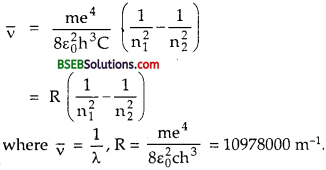For Paschen series, n1= 3, n2 = ∞ = for shortest wavelength. If λmin be the minimum wavelength in this series of spectral lines, then –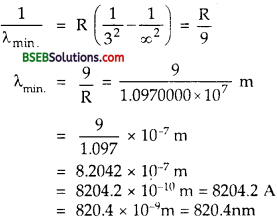Question 4.
A difference of 2.3 eV separates two energy levels in an atom. What is the frequency of radiation emitted when the atom make a transition from the upper level to the lower level?
Here, AE = 2.3 eV = 2.3 x 1.6 x 10-19 J.
h = 6.62 x 10-34JS.
v = frequency of radiation emitted = ?
Using the relation,Question 5.
The ground state energy of hydrogen atom is -13.6 eV. What is the kinetic and potential energies of the electron in this state?
We know that the K.E. of the electron in the nth orbit of hydrogen atom is given by
T = K.E = $$\frac {1}{2}$$ m vn2 = $$\frac{2 \pi^{2} \mathrm{k}^{2} \mathrm{me}^{4}}{\mathrm{n}^{2} \mathrm{~h}^{2}}$$ where K = $$\frac{1}{4 \pi \varepsilon_{0}}$$
for ground state, n = 1
∴ T = K.E = $$\frac{2 \pi^{2} \mathrm{k}^{2} \mathrm{me}^{4}}{\mathrm{~h}^{2}}$$ …………(1)
Also we know that the P.E. of the electron in the nth orbit of hydrogen atom is given by
V = P.E = – K $$\frac{\mathrm{e}^{2}}{\mathrm{r}_{\mathrm{n}}}$$ = – $$\frac{\mathrm{k}^{2} \cdot 4 \pi^{2} \mathrm{me}^{4}}{\mathrm{nh}^{2}}$$
∴ V = P.E. in ground state is given by
V = P.E = – 4π2K2 $$\frac{\mathrm{m} e^{4}}{\mathrm{~h}^{2}}$$ ………(2)

If E be the total energy in the ground state, then
E = K.E. + P.E. = T + V = $$\frac{-2 \pi^{2} k^{2} m e^{4}}{h^{2}}$$
= -T. ………..(3)
Here, E = Total energy in ground state = -13,6 eV. T = ?, V = ?
∴ From (3), T = – E = (- 13.6) = 13.6 eV.
and V = E – T = -T – T = – 2T
= – 2 x 13.6 = – 27.2 eV.
K.E. = 13.6 eV, P.E. = – 27.2 eVQuestion 6.
A hydrogen atom initially in the ground level absorbs a photon, which excites it to the n = 4 level. Determine the wavelength and frequency of photon.
We know that the energy En of the hydrogen atom in the nth stationary state is given by :
En = $$\frac{-13.6}{n^{2}}$$
For ground state, n = 1,
∴ En = E4
and for n = 4 level, En = En.
h = 6.62 x 10-34 Js.
Let λ and v be the wavelength and frequency of the photon absorbed by the hydrogen atom = ?
If E = hv be the energy absorbed, then
hv = E4 – E1 ……..(1)
Now E4 = $$\frac{-13.6}{4^{2}}=\frac{-13.6}{16}$$ = – 0.85 eV.
and Et = -13.6eV. ……(2)
∴ From (1) and (2), we get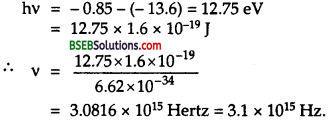Using relation
∴ C = vλ, we getQuestion 7.
(a) Using the Bohr’s model calculate the speed of the electron in a hydrogen atom in the n = 1,2, and 3 levels,
(b) Calculate the orbital period in each of these levels.
(a) According to Bohr’s model, we know that the radius of nth orbit and speed of electron in the nth orbit are given by :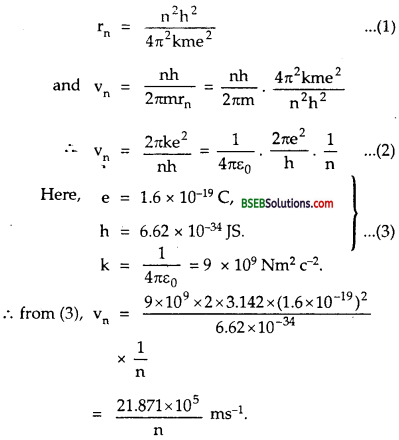Let v1, v2 and v3 be speeds of electron in the orbits n = 1,2 and 3.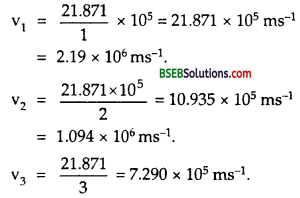(b) Let T1, T2 and T3 be the orbital time period in level no. 1,2 and 3 resepectively.
Now we know that time period in nth orbit is given by :
Tn = $$\frac{2 \pi \mathrm{r}_{\mathrm{n}}}{\mathrm{v}_{\mathrm{n}}}$$
Using eq (1) and (2), we getQuestion 8.
The radius of the innermost electron orbit of a hydrogen atom is 5.3 1011 m. What are the radii of the n = 2 and n = 3 orbits?
We know that the radius of the innermost orbit of electron in the hydrogen atom is given by :
rn = $$\frac{4 \pi \varepsilon_{0} n^{2} h^{2}}{4 \pi^{2} m e^{2}}$$ = Knn
where K = $$\frac{4 \pi \varepsilon_{0} \mathrm{~h}^{2}}{4 \pi^{2} \mathrm{me}^{2}}$$
where h = 6.62 x 10-34 Js, m = 9.1 x 10-31 kg, e = 1.6 x 10-19C.
for n = 1, rn = r1
r1 = 5.3 x 10-11m = K.
for n = 2,
r2 = KZ2 = 4r1 = 4 x 5.3 x 10-11 = 21.2 x 10-11m
= 2.12 x 10-10 m
for n = 3, r2 = K2 32 = 9 r2 = 9 x 5.3 x 10-11 H = 47.7 x 10-11 m
= 4.77 x 10-10 m.Question 9.
A 12.5 eV electron beam is used to bombard gaseous hydrogen at room temperature. What series of wavelengths will be emitted?
Here, E = energy of electron beam = 12.5 eV
= 12.5 x 1.6 x 10-19J.
h = 6.62 x 10-34JS
C = 3 x 108 ms-1
Let λ = wavelength of radiation emitted due to bombardment of electron beam = ?
Using the relation,
E = $$\frac{\mathrm{hC}}{\lambda}$$ , we get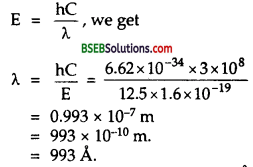This wavelength falls in the range of Lyman series (912 Å to 1216 Å), thus we conclude that Lyman series of wavelength 993 Å is emitted.

Question 10.
In accordance with the Bohr’s model, find the quantum number that characterises the earth’s revolution around the sun in an orbit of radius 1.5 x 1011 m with orbital speed 3 x 104 m/s. (Mass of earth = 6.0 x 1024 kg.)
Here, r = radius of orbit of earth around the sun = 1.5 x 1011
m = mass of earth = 6.0 x 1024 kg.
v = orbital speed of earth = 3 x 104 ms-1,
h = 6.62 x 10-34 JS.
n = quantum number of the orbit of earth around the sun in which it revolves around the sun = ?
Using the relation,The quantum number for the satellite motion is extremely large. In fact for such large quantum numbers, the results of quantisation conditions tends to those of classical physics i.e., the difference between the two successive energy or angular momentum levels is very small and the levels may be considered continuous.

Question 11.
Answer the following questions, which help you understand the difference between Thomson’s model and Rutherford’ model better.
(a) Is the average angle of deflection of a-particles by a thin gold foil predicted by Thomson’s model much less, about the same, or much greater than that predicted by Rutherford’s model?
(b) Is the probability of backward scattering (i.e., scattering of expat-tides at angles greater than 90°) predicted by Thomson’s model much less, about the same, or much greater than that predicted by Rutherford’s model?
(c) Keeping other factors fixed, it is found experimentally that for small thickness t, the number of a-particles scattered at moderate angles is proportional to t. What clue does this linear dependence on t provide?
(d) In which model is it completely wrong to ignore multiple scattering for the calculation of average angle of scattering of a- particles by a thin foil?
(a) The average angle of deflection of a-particles by a thin gold foil predicted by Thomson’s model is about the same as that predicted by Rutherford’s atom model. This is because we are talking of average angle of deflection.
(b) The probability of backward scattering predicted by Thomson’s model is much less than that predicted by Rutherford’s model. This is because there is no such massive central core called the nucleus as in Rutherford’s model.
(c) This linear dependence on suggests that scattering is predominantly due to a single collision, because chance of a single collision increases with the number of target atoms which increases linearly with the thickness of the foil.
(d) In Thomson model, positive charge is uniformly distributed in the spherical atom. Therefore, a single collision causes very little deflection Thus average scattering angle can be explained only by considering multiple scattering in Thomson model.
On the other hand, in Rutherford’s model, most of the scattering comes from a single collision. So multiple scattering may be ignored.Question 12.
The gravitational attraction between electron and proton in a hydrogen atom is weaker than the coulomb attraction by a factor of about 10’40. An alternative way of looking at this fact is to estimate the radius of the first Bohr orbit of a hydrogen atom if the electron and proton were bound by gravitational attraction. You will find the answer interesting.
Let mp and me be the masses of proton and electron respectively lying at a distance r.
If F be the gravitational force between them, then
F = G $$\frac{m_{e} m_{p}}{r^{2}}$$ …………(1)
This force provides the necessary centrepetal force for uniform circular motion :According to Bohr’s condition,
mevr = G $$\frac{\mathrm{nh}}{2 \pi}$$ ……….(4)
Squaring on both sides of (4) we get,
me2v2r2 = $$\frac{n^{2} h^{2}}{4 \pi^{2}}$$ ……….(5)
Dividing (5) by (3) we get.Also according to Bohr’s model, the radius of first orbit is given by
r1 = $$\frac{4 \pi \varepsilon_{0} \mathrm{~h}^{2}}{\mathrm{~m}_{\mathrm{e}} \mathrm{e}^{2}}$$ …………(7)
From (6) and (7), we conclude that if the place of electrostatic force (=$$\frac{e^{2}}{4 \pi \varepsilon_{0} r^{2}}$$) , We consider the atom bound by the gravitational force (= G = mpme/r2) then we should replace $$\frac{e^{2}}{4 \pi \varepsilon_{0}}$$ by G mp me. thus the radius of the first Bohr orbit in gravitional bound hydrogen atoms is given by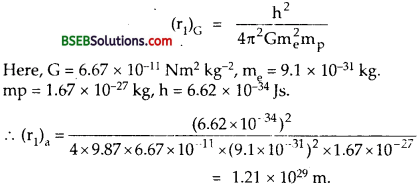Question 13.
Obtain an expression for the frequency of radiation emitted when a hydrogen atom de-excites from level n to level (n – 1). For large n, show that this frequency equals the classical frequency of revolution of the electron in the orbit.
The energy of an electron in the nth orbit of hydrogen atom is given by the relation,
E = $$-\frac{2 \pi^{2} m e^{4} k^{2}}{n^{2} h^{2}}$$ …………..(1)
Where K = $$\frac{1}{4 \pi \varepsilon_{0}}$$ is a constant.
Also the energy of an electron in the (n – 1) th orbit of hydrogen atom is given by the relation :
En – 1 = $$\frac{-2 \pi^{2} m e^{4} k^{2}}{(n-1)^{2} h^{2}}$$ ………..(2)
Thus if v be the frequency of the radiation emitted when the hydrogen atom de-excites from the nth level to (n – 1)th level, thenFor large value of n, (2n – 1) ≈ 2n and.
(n – I) ≈ n.
∴ From eq. (3), we getAlso according to Bohr’s atom model the velocity of electron in the nth orbit is :
υn = $$\frac{\mathrm{nh}}{2 \pi \mathrm{mr}}$$
and the radius of the nth orbit is given by :
r = $$\frac{n^{2} h^{2}}{4 \pi^{2} m k e^{2}}$$
∴ the classical Frequency of revolution of electron is given byFrom (4) and (5), we see that v = vc i.e., for large value of n, the classical frequency of revolution of electron in nth orbit is the same as the frequency of radiation emitted when the hydrogen atom de-excites from level (n) to level (n – 1). This is called Bohr’s Correspondence principle.

Question 14.
Classically, an electron can be in any orbit around the nucleus of an atom. Then what determines the typical atomic size? Why is an atom not, say, thousand times bigger than its typical size? The question had greatly puzzled Bohr before he arrived at his famous model of the atom that you have learnt in the text. To simulate what he might well have done before his discovery, let us play as follows with the basic constants of nature and see if we can get a quantity with the dimensions of length that is roughly equal to the known size of an atom (~ 10-10m).
(a) Construct a quantity with the dimensions of length from the fundamental constants e, me, and c. Determine its numerical value.(b) You will find that the length obtained in (a) is many orders of magnitude smaller than the atomic dimensions. Further, it involves c. But energies of atoms are mostly in non – relativistic domain where c is not expected to play any role. This is what may have suggested Bohr to discard c and look for ‘something else’ to get the right atomic size. Now, the Planck’s constant h had already made its appearance elsewhere. Bohr’s great insight lay in recognising that hr me, and e will yield the right atomic size. Construct a quantity with the dimension of length from h, me, and e and confirm that its numerical value has indeed the correct order of magnitude.
(a) Here, the dimensional formula of e is [AT],
The dimensional formula of C = [LT– 1]
The dimensional formula of me = [M]
The dimensional formula of $$\frac{1}{4 \pi \varepsilon_{0}}$$ = [ML3 T-4 A2]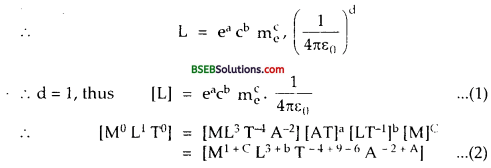∴Comparing dimensions of M1 L1T and A in equation (2), we get
M, 0 = 1 + C or C = $$\frac {-}{C}$$
L, 1 = 3 + b, ∴ b = 1 – 3 = – 2.
T,0 = -4 + 9 – b. ∴ a = 4 + b = 4 – 2 = 2
A, 0 = – 2 + A, ∴ A = 2.
Thus, eq (l), becomes :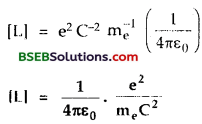Thus the quantity having the dimensions of length is $$\left[\frac{1}{4 \pi \varepsilon_{0}} \cdot \frac{\mathrm{e}^{2}}{\mathrm{~m}_{\mathrm{e}} \mathrm{C}^{2}}\right]$$
∴ numerical value of this quantity is –(here e = 1.6 x 1019 C, m = 9.1 x 1031, C = 3 x 108 ms-1).
Which is very small as compared to the size of the atom (= 10-10 m).

(b) Thus from (a), we see that the magnitude of this quantity is many orders of magnitude smaller than the atomic dimensions.
Let the quantity x constructed from h, me and e given byComparing Powers of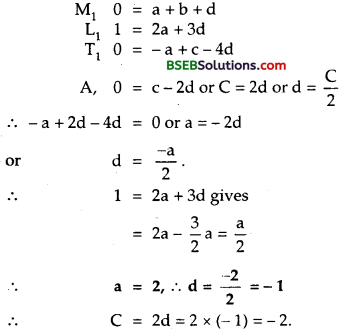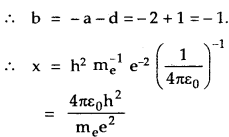is the required quantity having dimension of length. Its value is given by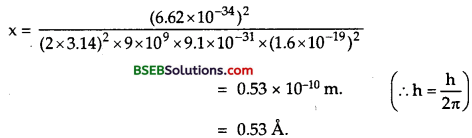Which is nearly equal to the size of the atom. Thus we confirm that its value is of the same order as the atomic size.

Question 15.
The total energy of an electron in the first excited state of the hydrogen atom is about – 3.4 eV.
(a) What is the kinetic energy of the electron in this state?
(b) What is the potential energy of the electron in this state?
(c) Which of the answers above would change if the choice of the zero of potential energy is charged?
Here, total energy in first excited state (i.efor n = 2) of H – atom, E = – 3.4 eV.
K.E of electron = K $$\frac{\mathrm{he}^{2}}{2 \mathrm{r}}$$, Where K = $$\frac{1}{4 \pi \varepsilon_{0}}$$
And P.E = – $$\frac{\mathrm{Ze}^{2}}{\mathrm{r}}$$ = -2 $$\left(\frac{\mathrm{k} Z \mathrm{e}^{2}}{2 \mathrm{r}}\right)$$
= – 2(K.E)
∴ E = Total energy in first excited state is given by
E = K.E. + P E, = K.E. + [- 2 (K.E.)] = – K,E,
Physics-XII (Part-II)
(a) ∴ K.E. = – E = – (- 3.4) = + 3.4 eV.
(b) P.E. = – 2 (K.E.) = – 2 x 3.4 = – 6.8 eV.
(c) If zero of the potential energy is changed, K.E. does not change and continues to be + 3.4 eV as it is independent of the choice of the zero of potential energy.
But the P.E. and total energy of the state would change with the choice of the zero of the potential energy.Question 16.
If Bohr’s quantisation postulate (angular momentum = $$\frac{\mathrm{nh}}{2 \pi}$$) is a basic law of nature, it should be equally valid for the case of planetary motion also. Why then do we never speak of quantisation of orbits of planets around the sun?
Applying Bohr’s quantisation postulate : we get
mvr = $$\frac{\mathrm{nh}}{2 \pi}$$
or n = mv x $$\frac{2 \pi}{\mathrm{h}}$$ …………(1)
For the motion of a planet (ray, earth) Let us take :
m = 6 x 1024 kg.
V = 3 x 104 kg.
r = 1.49 x 1011m.
h = 6.62 x 10-34 JS.
∴ From (1),
n = $$\frac{6 \times 10^{24} \times 30000 \times 1.49 \times 10^{11} \times 2 \times 3.14}{6.626 \times 10^{-14}}$$
= 2.49 x 1074
i.e., n is very large. So the angular momentum associated with planetary motion are incomparably large relateive to h. For such a large value of n, the differences in the successive energies and angular moments of the quantised levels of the Bohr model are so small compared to the energies and angular momentum respectively of the levels that the levels may be considered continuous.

Question 17.
Obtain the first Bohr’s radius and the ground state energy of a ‘muonic hydrogen atom’ (i.e. an atom in which a negatively charged muon (μ) of mass about 207 me orbits around a proton).
A muonic hydrogen is the atom in which a negatively charged muon of mass about 20 % me revolves around a proton.
i.e., mu = 207 me = 207 x 9.1 x 10-31 kg.
Also according to Bohr’s model, r ∝ $$\frac {1}{m}$$
Let ru = first Bohr’s radius of muonic hydrogen atom.
$$\frac{r_{\mu}}{r_{e}}=\frac{m_{e}}{m_{\mu}}=\frac{1}{207}$$ c
where re = radius of first orbit of the electron in hydrogen atom = 0.53 A =0.53 x 10-10 m.
∴ rμ = $$\frac{\mathrm{r}_{\mathrm{e}}}{207}=\frac{0.53 \times 10^{-10}}{207}$$ = 2.56 x 10-13 m-1
Let Eμ= ground state energy of a muonic hydrogen atom = ?
Also according to Bohr’s model,
E ∝ m.Where Ee = ground state energy of electron in the hydrogen atom = – 13.6 eV.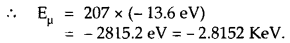Aliter :and energy olihis level is gken by –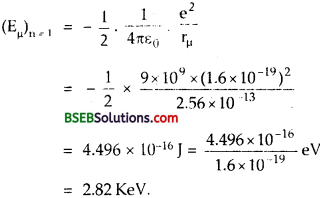### Bihar Board Class 12 Physics Atoms Additional Important Questions and Answers

Question 1.
State Bohr’s frequency condition.
It states that the frequency v of a radiation emitted on jumping of an electron from the outer orbit to the inner orbit of an atom is given by
E = hv = E2 – E1
Where E1 = total energy of the electron in the inner orbit and E2 = total energy of the electron in the outer orbit.

Question 2.
Define distance of closest approach.
It is defined as the minimum distance from the centre of nucleus upto which an energetic a-particle travelling directly towards the nucleus can move during head-on-collision before coming to rest and retracing its path. It is denoted by r0.

Question 3.
Define Impact parameter.
It is defined as the perpendicular distance of the velocity vector of the a-particle from the centre of the nucleus when it is far away from the atom. It is denoted by b.

Question 4.
Define energy level.
It is defined as the horizontal line which represents the total energy of an electron in a stationary orbit of an atom.

Question 5.
How an energy level is represented?
An energy level is represented by a horizontal line drawn according to some suitable energy scale.

Question 6.
Name the series of hydrogen spectrum which lies in the visible region of electromagnetic spectrum.
Balmer series of hydrogen spectrum lies in the visible region of electromagnetic spectrum.

Question 7.
Can a hydrogen atom absorb a photon whose energy exceed s its binding energy?
Yes, a hydrogen atom can absorb a photon whose energy exceeds its binding energy, but the atom would be ionised.

Question 8.
Name a physical quantity whose dimensions are the same as that of Plank’s constant.
Angular momentum has the same dimensions as that of Plank’s constant (= h).

Question 9.
Write an empirical relation for Paschen series lines of hydrogen atom.
$$\frac{1}{\lambda}=\bar{v}=R\left(\frac{1}{3^{2}}-\frac{1}{n^{2}}\right)$$
Where n = 4,5,6, ………..
and R = $$\frac{2 \pi^{2} m k^{2} e^{4}}{c h^{3}}$$ is called Rydberg constant and $$\frac{1}{λ}$$ v is
Called wave numder, K = $$\frac{1}{4 \pi \varepsilon_{0}}$$Question 10.
Which series of hydrogen atom lies in the U.V. region?
Lyman series of hydrogen atom líes in the U.V. region.

Question 11.
What ¡s the difference between an a-particle and a helium atom?
a-particle is only the nucleus of helium atom ie. a particle is He atom stripped off two electrons.

Question 12.
What does Rutherford’s scattering experiment prove?
Rutherford’s scattering experiment proves that the atom consists of a tiny central core in which the whole of the positive charge and nearly whole
mass of the atom is concentrated.

Question 13.
What is the physical significance of impact parameter?
It helps us in knowing the angle of scattering. Smaller the impact parameter, the larger is the electrostatic force, thus the scattering is also large. When the impaci parameter is large, the electrostatic force is weak, lhu angle of scattering O ola particle is also small.

Question 14.
Name the particle, the scattering of which can estimate the size of the nucleus?
a-particle scattering can estimate the size of nucleus.

Question 15.
The wavelengths of some of the spectral lines obtained in hydrogen spectrum are 9546 A, 6463 A and 1216 A. Which one of these wavelength belongs to Lyman series ?
The Lyman series lies in the ultraviolet region. So the wavelength of any line in the lyman series has to be less than the wavelength in the visible region i.e., less than 3900 A. Thus clearly 1216 A belongs to Lyman series.

Question 16.
The energy levels of an atom are shown in figure. Which one of these transitions results in the emission of a photon of wavelength 275 nm ?
Here, λ = 275 nm
= 275 x 10-9mThus the energy of this photon is given by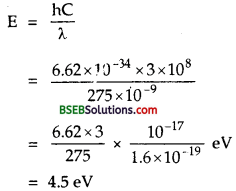Thus, the transition B [0 – (4.5)] = 4.5 eV is the required transition.

Question 17.
The potential energy of an electron in Bohr’s theory of hydrogen atom is negative. Moreover, its numerical value is greater than the kinetic energy. What is the physical significance of these facts?
The negative value of potential energy means that the electron is bound to the nucleus. The greater numerical value of potential energy implies that the electron cannot leave the atom on its own. It would require a supply of external energy for doing so.Question 18.
Why are Bohr’s orbits called “Stationary orbits”?
Bohr’s orbits are called “stationary orbits” because the electron can neither radiate nor absorb energy while revolving in an orbit.

Question 19.
The ionisation potential of helium atom is 24.6 V. How much energy will be required to ionise it?
The energy required to ionise helium atom will be 24.6 eV.

Question 20.
Why the mass of the nucleus has no significance in scattering in Rutherford’s experiment?
The cause of scattering in Rutherford’s experiment is the charge on the nucleus. The scattering occurs due to the electric field of the charge on the nucleus. So scattering is independent of the mass of the nucleus.

Question 21.
What path should an electron follow in Rutherford’s model? Use classical electromagnetic theory.
According to classical electromagnetic theory, an accelerated charge should emit electromagnetic radiation. Thus the energy and radius should also continuously go on decreasing. So the electron shall follow a spiral path and finally fall into the nucleus.

Question 22.
What would happen, if the electrons in an atom were stationary?
If the electrons in the atom were stationary, they would have been attracted by the nucleus due to coulomb’s attractive force and the atom would not have existed.

Question 23.
It is defined as the radius of the first orbit of hydrogen atom and is equal to 5.3 x 1011 m.

Question 24.
The speed of electron in the first orbit of hydrogen atom is 2 x 106 ms-1. What is the speed of the electron in the same orbit of He+ atom?
Here, vH = 2 x 106 ms-1; Z of He = 2.
Also we know that speed of electron in any orbit is directly proportional to Z.
∴ vHe = ZυH = 2 x 2 x 106 – 4 x 106 ms-1

Question 25.
The number of a particles scattered at moderate angles is proportional to a small thickness t. What does this suggest?
It shows that the scattering is msintly due to single collision. Celarly chances of collisions increase with the increase with the increae of target atoms with the increase in thickness of the soil.

Question 26.
When impact perimeter of a particle is zero, the a particle travelling directly towards the centre of the nucleus rettraces its path. Explain why?
We know that impact perimeter ‘b’ is given by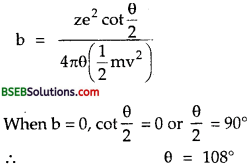Question 27.
Most mass of the atom is with positive charge. In a hydrogen atom, what fraction of atomic mass is with positve charge?
A hydrogen atom is made up of one proton and one electron and the mass of proton is 1836 times the mass of electronThus the fraction of the atomic mass with the positive charge is $$\frac{1836}{1837}$$

Question 28.
State Bohr’s quantisation condition of angular momentum. What is its value for electron in the second orbit?
It states that the angular momentum of an electron is integral multiple of $$\frac{\mathrm{h}}{2 \pi}$$
i.e., L = n $$\frac{\mathrm{h}}{2 \pi}$$ where n = 1,2,3 ………… for second orbit n = 2
∴ L = $$\frac{2 \mathrm{~h}}{2 \pi}=\frac{6.62 \times 10^{-34}}{2 \times 3.14}$$ = 2.11 x 10-34JsQuestion 34.
What do you mean by ground state energy?
It is defined as the lowest energy possible for an electron of an atom. It is the energy for which the principal quantum number is one.

Question 35.
What do you conclude if the total energy of the electron is:
(a) positive,
(b) negative?
(a) From positive value of total energy we conclude that the electron besides being free of the nucleus has kinetic energy also.
(b) From negative value of total energy we conclude that the electron is bound to the nucleus.

Question 36.
When is a monochromatic line emitted in the Balmer series of hydrogen atom?
A monochromatic line is emitted in the Balmer series of hydrgoen atom when the electron transits from an outer orbit (n = 3) to second orbit (n = 2).

Question 37.
Only certain lines are found in the atomic spectrum of the substances. Why?
This is because the atom has only certain energy state’s and hence only certain definite frequencies of radiation emitted by it are possible.

Question 38.
Why is that the mass of the nucleus does not enter in the formula for impact parameter but the charge does?
The scattering takes place due to the electrostatic field of the nucleus and for this reason, the charge of the nucleus enters into the expression for impact parameter.

Question 39.
State the limitations of Rutherford’s model of atom.
(i) It failed to explain the stability of the atom.
(ii) It failed to explain the spectrum of an atom.

Question 40.
Is there any relation between K.E. and P.E. of an electron moving in a circular path of radius rn with a speed un around the nucleus.
Yes, the K.E. is half of the P.E.

Question 1.
What were the observations drawn by Geiger and associates from a-particle scattering experiment?
Following are the observations drawn from a-particle scattering experiment:
(i) Most of the a-particles passed through the gold foil undeflected.
(ii) Few a-particles were deflected through small angles.
(iii) Some a-particles weredeflected through large angles (> 90°).
(iv) Very few a-particles suffered a deflection of 180°.
(v) The number of a-particles per unit area that reach the screen at a scattering angle θ were found to vary as :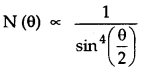Question 2.
Derive the expression for the distance of closest appraoch.
Let an α-particle having energy E $$\left(=\frac{1}{2} \mathrm{mv}^{2}\right)$$ MeV is directed towards the centre of nucleus. The force of repulsion between the nucleus and the a-particle increases as it moves towards thenucleus and thus its velocity goes on decreasing. When the distance between α-particles and the nucleus is equal to the distance of closest approach (r0), then the a-particle comes to rest and at this point, the K.E. of α- particle is corhpletely converted into electric potential energy of the system.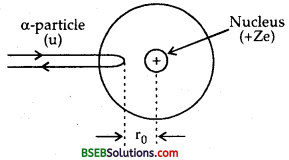K.E of α-particle, E = $$\frac {1}{2}$$ mv2 ………..(1)
and P.E. of the system = electric potential at r0 due to nucleus x charge on the α-particle.Which is the required expression for r0.

Question 3.
What are the limitations of Bohr’s atomic model?

• It could successfully explain the spectra of simple atoms having one electron but it could not explain the spectra of comple atoms having more than one electron.
• It does not give any idea about the distribution of electrons in an atom.
• This model could not account for the wave nature of electrons.
• It does not give any idea about the relative intensity of spectral lines.
• It could not explain the splitting of spectral lines into a number of spectral lines under the effect of magnetic field.

Question 4.
Derive the expression for speed of an electron in the nth orbit.
We know that
$$\frac{m v_{n}^{2}}{r_{n}}=k \frac{Z e^{2}}{r_{n}^{2}}$$
or mv22rn = k Ze2 ………(i)
Also we know that, mvn rn = $$\frac{\mathrm{nh}}{2 \pi}$$ ………..(2)
∴ From (1) and (2), we getWhich is the required expression for speed of an electron in nth orbit.

Question 5.
State energy quantization.
It states that the energy of hydrogen atom can have only some definite values as the principal quantum number n can definite integral values n = 1, 2, 3 …………
These definite possible values of energy show that energy : quantized in the hydrogen atom.Question 6.
Define excitation energy. Derive the expression for the energy required to excite an atom from one energy Level to the another level.
It is defined as the energy required to excite an electron from the lowest energy level i.e., ground state to any higher energy state.
Expression : Let E1 and E2 be the energy of an electron in the ground state and 1st excited state respectively.
If E be the amount of energy to be supplied, then
E = E2 – E1Question 7.
Derive the expression for the frequency of radiation emitted by an H-atom when an electron jumps from higher energy to lower energy level.
Let an electron jumps from higher energy level n2 to lower energy level n1 Let E2 and E1 be the energies of these levels. If hv be the energy of the atom emitted, then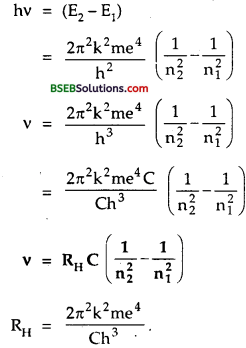Question 8.
Draw energy level diagram of hydrogen atom indicating the enrgy associated with each orbit.
We know that the energy of an electron in the nth orbit of hydrogen atom is given by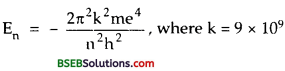For 2nd orbit, n = 1, ∴E1 = – 13.6 eV
For 2nd orbit, n = 2, ∴ E2 = $$\frac{m v_{n}^{2}}{r_{n}}=k \frac{Z e^{2}}{r_{n}^{2}}$$ = – 3.4 eV.
For 3rd orbit, n = 3, ∴ E3 = $$\frac{m v_{n}^{2}}{r_{n}}=k \frac{Z e^{2}}{r_{n}^{2}}$$ = – 1.51 eV.
For 4th orbit, n = 4, ∴ E4 = $$\frac{m v_{n}^{2}}{r_{n}}=k \frac{Z e^{2}}{r_{n}^{2}}$$ = – 0.85 eV.
The energy level diagram is shown in the following figure.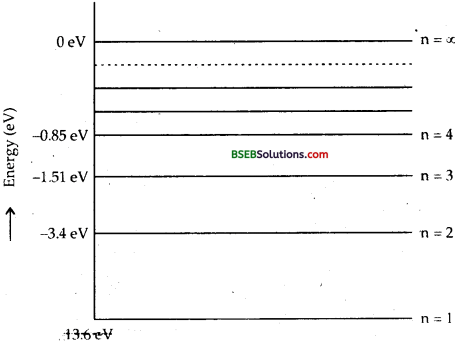Question 9.
Define ionisation energy of an atom.
It is defined as the energy required to remove an electron from its ground state to completely out of the atom.
i,e Ionisation energy of H-atom = E – E1
= 0 – ( – 13.6) = 13.6 eV.

Question 10.
Why we obtain a number of lines in the emission spectrum of hydrogen atom while it has only one electron?
This is because there are number of allowed energy orbits. When the electron jumps from higher to a lower orbit, we get the emission spectrum. The electron can jump successfully from one higher energy orbit to lower energy orbit. Also there are large number of atoms in sample of hydrogen.

Question 1.
Express emission of various series of spectral lines in case of hydrogen atom.
When an electron jumps from higher orbit n; to lower orbit nf in case of H-atom (Z = 1), the frequency of radiations emitted is given by
$$\bar{v}=R_{H}\left(\frac{1}{n_{f}^{2}}-\frac{1}{n_{i}^{2}}\right)$$
It shows that the radiations emitted by the excited hydrogen atom must contain well defined series of wavelengths depending upon the quantum numbers n and nf. There are the following series of spectral lines in case of H-atom. (i) Lyman Series – It is the series in which the spectral lines correspond to the transition of electron from some higher energy states to the lower energy state nf = 1.
∴ $$\bar{v}=\frac{1}{\lambda}=R_{H}\left(\frac{1}{1^{2}}-\frac{1}{n_{i}^{2}}\right)$$
where ni = 2,3,4,……….
They lie in UV region of the spectra.(ii) Balmer series – It is the series in which spectral lines correspond to the transition of electron from some higher energy states to the lower energy state nf = 2.
i.e $$\bar{v}=\frac{1}{\lambda}=\hat{R}_{H}\left(\frac{1}{Z^{2}} \-\frac{1}{n_{i}^{2}}\right)$$
Where ni = 3,4,5………
These lie in the visible region of spectra.

(iii) Paschen series – It is the series in which sjvi’jal lines correspond to the transition of electron from some higher ene. gy states to the lower energy state nf= 3.
i.e $$\bar{v}=\frac{1}{\lambda}=\mathrm{R}_{\mathrm{H}}\left(\frac{1}{3^{2}}-\frac{1}{\mathrm{n}_{\mathrm{i}}^{2}}\right)$$
where ni = 4,5,6,……….
These lie in the 1 R region of spectra.

(iv) Brackett series – It is the series obtained by the transition of electron from some higher energy states to the lower energy state nf = 4These lie in the infrared region of spectra.

(v) Pfund series – It is the series obtained by the transition of electron from some higher energy state nf = 5, i.e. nf = 5, ni = 6, 7,8 …….
∴ $$\bar{v}=\frac{1}{\lambda}=R_{H}\left(\frac{1}{5^{2}}-\frac{1}{n_{i}^{2}}\right)$$
These lie in IR region of spectra.

Question 2.
Derive the expression for total energy of an electron revolving in nth orbit of an atom.
Total energy of an electron in any orbit is the sum of the K.E. and P.E.
Let rn – radius’of nth orbit of the electron.
vn = speed of electron in nth orbit.
Ze = charge on the nucleus of the atom
m = mass of the electron
e = charge of the electron.
∴ its K.E. is given by
K.E = $$\frac {1}{2}$$ mvn2 ………..(1)
Also we knoe that
υn = $$\frac{2 \pi \mathrm{k} \mathrm{Ze}^{2}}{\mathrm{nh}}$$ ……….(2)
∴ From (1) and (2), we get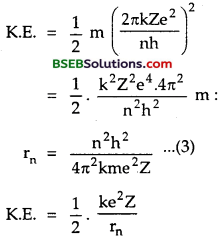P.E. of electron in the orbit of radius rn is given byIf En be the total energy of electron, then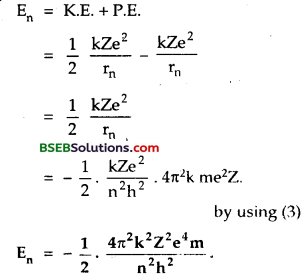Question 3.
State the basic postulates of Bohr’s model of hydrogen atom. Derive an expression for the stationary energy levels of a hydrogen atom.
Postulates of Bohr’s atom model – The following are the assumptions made by Bohr to explain the stability and spectrum of the atom. There are called postulates :
(i) An atom has a small positively charged core where whole of the mass of an atom is supposed to be concentrated and it is called nucleus of the atom.
The electrons revolve round the nucleus in fixed orbits of definite radii without radiating energy. There orbits are called stationary orbits or non-radiating orbits. There orbits are also called permitted orbits.2. The electrons revolve round the nucleus only in those orbits in which its angular momentum is an integral multiple of $$\frac {h}{2π}$$ .
i.e L = mvr = n $$\frac {h}{2π}$$ ……..(1)
equation (1) is known as quantisation condition of angular momentum.

(3) The energy is radiated in the form of a photon only when an electron jumps from higher energy orbit to lower energy orbit. The amount of energy readiated in the form of a photon is given by
Ei – Ef = hv.
where Ei and Ef are the energy associated with the orbits having principal quantum numbers ni and nf respectively (ni > nf).

Expression for stationary energy levels :
Let us consider Ze as charge an atomic nucleus. Let rn be the radius of orbit in which the electron revolves with a speed vn.
m = mass of electron. According to Bohr’s first postulate, The necessary centrepetal force is provided by force of attraction between the electron and the nucleus i.e.,
$$\frac{m v_{n}^{2}}{r_{n}}=\frac{1}{4 \pi \varepsilon_{0}} \cdot \frac{(Z e) e}{r_{n}^{2}}=\frac{h Z e^{2}}{r_{n}^{2}}$$
for H-atom, Z = 1
∴mvn 2 = $$\frac{\mathrm{ke}^{2}}{\mathrm{r}_{\mathrm{n}}}$$ …………(1)

Also according to Bohr’s second postulate,
mvnrn = $$\frac{\mathrm{nh}}{2 \pi}$$
or vn = $$\frac{\mathrm{nh}}{2 \pi}$$ ……..(2)
∴ From (1) and (2), we getWhich is the required expression for stationary orbits i.e., for their radii.
Clearly rn ∝ n2.
i.e., radii of stationary orbits are in the ratio of 12 : n2 : 32 : 42………

Numerical Problems

Question 1.
Calculate the impact parameter of a 5 MeV a-particle scattered by 10°, when it approaches a gold nucleus (Z = 79).
Here, Z = 79
θ = angle of scattering of a-particle
= 10°
b = impact parameter = ?

K.E of α-practicle = $$\frac {1}{2}$$mv2 = 5 Mev
= 5 x 106 x 1.6 x 10-19JS
= 8 x 10-13J
e = 1.6 x 10-19 C

Using the relation,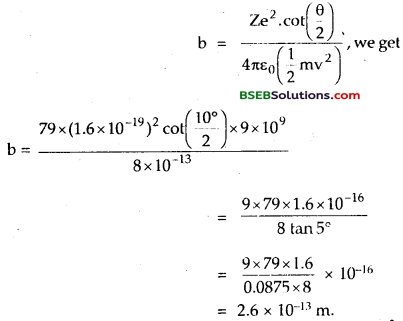Question 2.
The wavelength of Kα line (or copper is 1.36 Å. Calculate the ionisation potential of a K-shell electron in copper.
Here, A of Kα line = 1.36 Å = 1.36 x 10-10 m
V = ionisation potential =?
For Kα line, n1 = t, n2 = 2.
∴ Using the relation,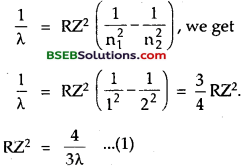The ionisation energy of K-shell electron is given by∴ From (1) and (2), we get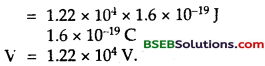Question 3.
If elements principal quantum number n > 4 did not exist in nature, what would be the possible number of electrons?
Up to quantum number 4, an atom has K, L, M and N shells. In any shell, maximum number of electrons is 2 n2,
∴Maximum number of electrons in K-shell = 2 x 12 = 2.
Maximum number of ele trons in L-shell = 2 x 22 = 8
Maximum number of electrons in M-shell = 2 x 32 = 18
Maximum number of electrons in N-shell = 2 x 42 = 32.
∴ Total number of maximum electrones in these shells = 2 + 8 + 18 + 32 = 60.
∴ Possible number of electrons would be 60.Question 4.
The wavelength of first member of Lyman series is 1216 A. Calculate the wavelength of 3rd member of Paschen series.
We know that $$\frac{1}{\lambda}=\mathrm{R}\left(\frac{1}{\mathrm{n}_{1}^{2}}-\frac{1}{\mathrm{n}_{2}^{2}}\right)$$
For frist member of Lyman series,
n1 = 1, n1 = 2,
∴ $$\frac{1}{\lambda}=\mathrm{R}\left(\frac{1}{1^{2}}-\frac{1}{2^{2}}\right)=\frac{3}{4} \mathrm{R}$$
R = $$\frac{4}{3 \lambda}$$ ……….(2)
Where λ = 1216 Å = 1216 x 10-10 m.
For 3rd member of Paschen series, n1 = 3, n2 = 6.
Let λ’ be the wavelength of 3rd member of Paschen series = ?
∴ Using (1), we get ,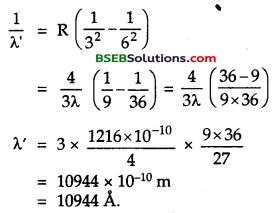Question 5.
The wavelength of Hβ line of Balmer series is 4861 A. Calculate the wavelength of Ha line of the series.
Here, for Hp line, λ’ = 4861 Å = 4861 x 10-10 m.
n1 = 2, n2 = 4.
for tire line, n1 = 3, n2 = 3.
Let λ be the wavelength emitted.
∴ Using the relation,
$$\frac{1}{\lambda}=\mathrm{R}\left(\frac{1}{\mathrm{n}_{1}^{2}}-\frac{1}{\mathrm{n}_{2}^{2}}\right)$$, we get
for Hβ line $$\frac{1}{\lambda}=\mathrm{R}\left(\frac{1}{\mathrm{2}_{1}^{2}}-\frac{1}{\mathrm{4}_{2}^{2}}\right)$$
= $$R \frac{(4-1)}{16}=\frac{3}{16}$$R
R = $$\frac{16}{3 \lambda^{\prime}}$$
For Hα line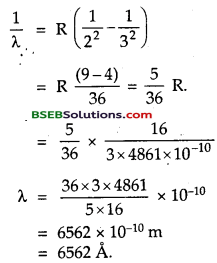Question 6.
Hydrogen atom in its ground state is excited of means of monochromatic radiation of wavelength 975 A. How many lines are pissible in the resulting emission spectrum? Calculate the longest wavelength amongst them. You may assume the ionisation energy for hydrogen atom as 13.6 eV. Take h = 6.63 x 10-34JS,
C = 3 X 108 ms-1.
Here, λ = Wavelength of monochromatic light = 975 Å = 975 x 10-10m.
(I.E.)H = ionisation energy = 13.6 eV of H-atom
C = 3 x 108 ms-1
h = 6.63 x 10-34JS.
Let E’ be the energy given by radiation of wavelength λ = 975 Å to the Hydrogen atom.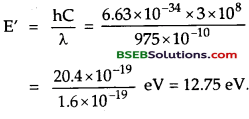Also energy of H – atom in the ground state is given by
E = – (I.E.)H = – 13.6 eV.
Let the atom gets excited to some n level after absorbing radiation of energy 12.75 eV.
If En be the energy of nth level, then
En = E + E’ = – 13.6 eV + 12.75 eV = – 0.85 eV.
Also wo know that c E -13.6i.e., the electron of the atom is excited to fourth energy level end it can return to any of the lower energy levels as shown in the figure here.Thus 6 lines will b possible in the emission spectrum.
The longest wavelenth will correspond to the emission between the closest energy levels le., n =4 and n =3.
The minimum energy difference between the closest levt is :
∆E = E4 – E3 = – 0.85 – (- 1.51) = 0.66eV.
= 0.66 x 1.6 x 10-19 J
= 1.056 x 10-19 J

∴ longest wavelength emitted is given by λ = $$\frac {hC}{∆E}$$
= $$\frac{6.63 \times 10^{-34} \times 3 \times 10^{8}}{1.056 \times 10^{-19} \mathrm{~J}}$$
= l8835 x 10-10m
= 18835 Å.Question 7.
Electrons of energies 10.2 eV and 12.09 eV can cause radiation to be emitted from hydrogen atom. Calculate In each case, the principal quantum number of the orbit to which the electron in the hydrogen atom is raised and the wavelength of the radiation emitted ¡fit drops back to the giound state.
In H-atom, the energy of the electron in the nih energy level is
En = $$-\frac{13.6}{n^{2}}$$ eV
for n = 1,
E1 = – 13.6eV
for n = 2,
E2 = $$\frac{-13.6}{4}$$ = -3.4eV
for n = 3,
E3 = $$\frac{-13.6}{4}$$ = -1.5leV.
∴ ∆E = E2 – E2 = -3.4 – (-13.6) = 10.2eV.
and E3 – E1 = -1.51 eV – (-13.6) = 12.09 eV.

∴ The çlectron in the hydrogen atom is raised to the states having principal quantum numbers 2 and 3 corresponding to radiation energies 10.2 eV and 12.09 eV respectively.
Let λ1 and λ2 be the wavelength of radiation emitted.
∴ λ1 = $$\frac{hC}{∆E}$$
= $$\frac{6.62 \times 10^{-34} \times 3 \times 10^{8}}{10.2 \times 1.6 \times 10^{-19}}$$
= 1.216 x 10-7m
= 1.216 x 10-10m = 1216 Å

similary
λ2 = $$\frac{6.62 \times 10^{-34} \times 3 \times 10^{8}}{12.09 \times 1.6 \times 10^{-19}}$$
= 1.026 x 10-7 m
= 1.026 x 10-10m
= 1026 Å

Question 8.
The stopping potential for the photo electrons emitted from a metal surface of work-function 1.7 eV is 10.4 volt. Find the wavelength of radiation used. Also identify the energy levels in the hydrogen atom which will emit this wavelength.
Here, W = Work function = 1.7 eV. = 1.7 x 1.6 x 10-19 J.
V0 = Stooping potential = 10.4 V.
h = 6.63 x 10-34JS
C = 3 x 108 ms-1
e = 1.6 x 10-19 C
λ = wavelength = ?
according to Einsteins photoelectric equation,The energy levels in the hydrogen atom which will emit the same wavelength are those whose energies differ by 12.1 eV. Now, energies in different levels of hydrogen atom are given by
E 1 = -13.6eV,
E2 = $$\frac{13.6}{4}$$ = – 3.4eV
E3 = $$\frac{-13.6}{9}$$ eV = -1.51eV
∴ ∆E = E3 – E1 = -15.1 – (- 13.6) = 12.1 eV
Thus required energy levels in the hydrogen atom are ground level (n = 1) and second excited level (n = 3).Question 9.
A p meson (charge = – e, mass = 207 m, where m is the mass of electron) can be captured by a proton to form a hydrogenlike mesic atom. Calculate the radius of first orbit, the binding energy and the wavelength of the first line in the Lyman series for such an atom. The mass of the proton is 1836 times the mass of electron. The radius of 1st Bohr orbit and the binding energy of hydrogen are 0.53 A and 14.6 eV respectively, R = 109737 cm-1.
Here, m1 = mass of μ meson = 207 m.
m2 = mass of proton = 1836 m.
$$\frac{\varepsilon_{0} \mathrm{~h}^{2}}{\pi \mathrm{me}^{2}}$$ = radius of 1st Bohr orbit = 0.53 Å.
B.E = 14.6 eV = 14.6 x 1.6 x 10-19J.
Let μ = reduced mass of the system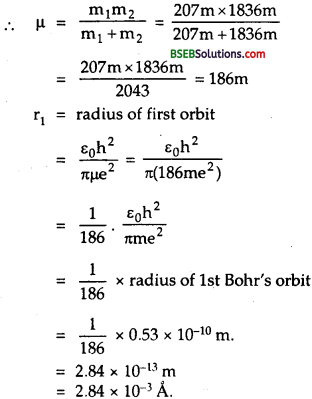From Bohr’s Theory, the ground state energy for hydrogen like atom with Z = 1, is given byThe wavelength of the Lyman lines are given by
$$\frac{1}{\lambda}=R_{M}\left(\frac{1}{1^{2}}-\frac{1}{n^{2}}\right)$$ n = 2,3,4………..
Where RM = Rydberg constant for mesic atom. For 1st line,Question 10.
With what energy an a-particle be directed towards the gold nucleus (Z = 72) so that it may retrace its path from a point which is 3 x 10-14 m away from the gold nucleus?
Here, r0 = distance of closest approach = 3 x 10-14 m.
Z = 72
E = $$\frac {1}{2}$$mv2 = K.E. of a-particle = ?
e = 1.6 x 10-19 C.

Using the relation,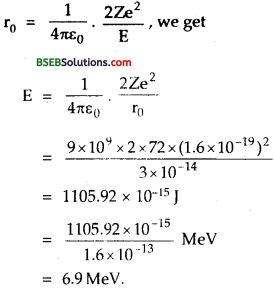Question 11.
An cl-particle having energy lO MeV travelling directly towards the nucleus of an atom is deflected back when its distance from the nucleus is 4 x 10-14 m. Calculate the atomic number of the atom.
Here, r0 4 x 10-14 m.
E = 10 MeV= 10 x l.6 x 10-13J
e = l.6 x 10-19C.
Let Z = atomic no. ol the nucleus.
∴Using the relation,Question 12.
(a) Which state of a tripply ionized beryllium atom (Be+++) has the same orbital radius as that of the ground state of hydrogen atom? ‘
(b) Compare the energies of the two states.
(a) We know that the radius of nth orbit of hydrogen atom is :
rn = $$\frac{n^{2} h^{2}}{4 \pi^{2} k m e^{2}}$$
for ground state, n= 1
∴ r1 = $$\frac{h^{2}}{4 \pi^{2} \mathrm{kme}^{2}}$$ ……….(1)
for hydrogen like atom, the radius of nth orbit is given by :
rn = $$\frac{1}{Z} \cdot \frac{n^{2} h^{2}}{4 \pi^{2} k m e^{2}}$$
for Be+++, Z = 4
rn = $$\frac{1}{4} \cdot \frac{n^{2} h^{2}}{4 \pi^{2} k m e^{2}}$$ ………..(2)
Now as rn = r1
∴ $$\frac{1}{4} \cdot \frac{n^{2} h^{2}}{4 \pi^{2} k m e^{2}}$$ = $$\frac{h^{2}}{4 \pi^{2} k m e^{2}}$$
or n1 = 4
or n = 2.
i.e. the 2nd orbit of Be+++ atom will have the same radius as that of the ground state of hydrogen atom.(b) The energy of an electron in the orbit fo 11- atom is given by”
En = $$\frac{2 \pi^{2} k^{2} m e^{4}}{n^{2} h^{2}}$$
for ground state, n = 1,
E1 = – $$\frac{2 \pi^{2} k^{2} m e^{4}}{h^{2}}$$ ………..(3)
and for hydrogen like atom, the energy of an electron in nth orbit is
En = $$-Z^{2} \frac{2 \pi^{2} k^{2} m e^{4}}{n^{2} h^{2}}$$
for Be+++, Z = 4, and n = 2.
E2 = $$-4^{2} \cdot \frac{2 \pi^{2} k^{2} m e^{4}}{2^{2} h^{2}}$$ ……….(4)
∴From (3) and (4), we get
E2 = 4E1
or $$\frac{\mathrm{E}_{2}}{\mathrm{E}_{1}}$$ = 4

Question 13.
Show that the velocity of electron in first orbit of hydrogen atom is times the velocity of light in free space.
Here, Z = 1, n = 1, C = 3 x 108 ms-1.
Orbital velocity of electron in nth orbit is given by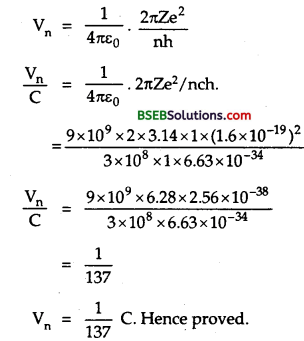Question 14.
A proton moves with a speed of 7.45 x 105 ms-1 directly towards a free proton originally at rest. Find the distance of closest approach for two protons.
Let v = speed of an electron moving towards a free proton at rest = 7.45 x 105 ms-1. They apply a force of mutual repulsion on each other due to which the stationary proton also starts moving. When at a distance of closest approach rQ, let both protons are moving with a velocity v’.
Applying the law of conservation of momentum, we get
mv = mv’ + mv’
= 2 mv’
V’ = $$\frac{v}}{2}$$ ……….(1)
Also according to law of conservation of energy,Question 15.
Determine the radius of the first orbit of the hydrogen atom. What would be the velocity and frequency of the electron in the first orbit? Given h = 6.62 x 10-34JS, m = 9.1 x 10-31M kg, e = 1.6 x 10-19C
Here, h = 6.62 x 10-34 JS,
m = 9.1 x 10-31 kg
e = l.6 x 10-19C
$$\frac{1}{4 \pi \varepsilon_{0}}$$ = 9 x 109 Nm2C2.
r1 = ?, v1 = ?, v1 =?
The radius of the nth orbit of hydrogen atom is given by
rn = $$4 \pi \varepsilon_{0} \frac{n^{2} h^{2}}{4 \pi^{2} m e^{2}}$$
for first orbit, n = 1.
r1 = $$4 \pi \varepsilon_{0} \frac{n^{2} h^{2}}{4 \pi^{2} m e^{2}}$$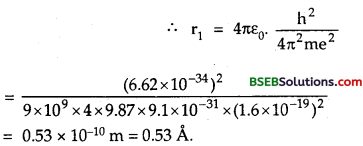v1 = Velocity of electron in first orbit =?
Velocity of electron in nth orbit is given by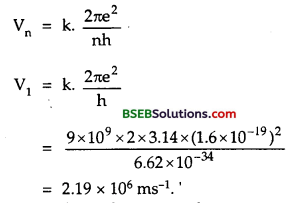∴v1 = frequency of electron in first orbit is given byQuestion 16.
Calculate the ionisation potential of hydrogen atom.
We know that
W = k2 $$\frac{2 \pi^{2} m e^{4}}{h^{2}}\left(\frac{1}{n_{1}^{2}}-\frac{1}{n_{2}^{2}}\right)$$
Ionisation potential is the energy required to remove an electron from ground state to infinity.
∴Here n1= 1, n2 = ∞Question 17.
The ground state energy of an electron in hydrogen atom is -13.6 eV. Find the energy of second excited state of Li++.
We know that for one electron atom, the energy of nth state is given by
En = $$\frac{13.6}{n^{2}}$$
For Li++ Z = 3 and 2nd excited state = -3.4 eV
n = 2 for 1st excited state
Eground state = ?
We know that for hydrogen atom, the energy in the nth state i given by
En = $$\frac{\mathrm{E}_{\text {ground state }}}{\mathrm{n}^{2}}$$
Eground state = n2.En = 22 x E2 = 4 x (-3.4) = -13.6 eVQuestion 19.
The number of a-particles scattered from a target at 60° is 4 x 106. What is the number of particles scattered at angle 90° under identical conditions?
We know that the number of particles scattered at angle ∞ is related to θ as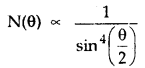Here, θ1, = 60°, N1, (θ1) = 4 x 106, θ1 = 90°, N22) = ?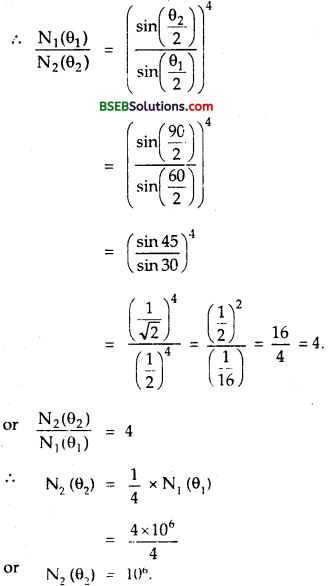Fill In The Blanks

Question 1.
………….. of the electron in the orbit signifies that the electron and nucleus is a bound system.
Negative energy.

Question 2.
The ………….. lies in the infrared region of the spectrum.
Paschen series.

Question 3.
Lyman series lies in the region of spectrum and Balmer series lies in the ………….. of the spectrum.
Ultraviolet, visible region.

Question 4.
The difference of energy levels goes on ………….. as we move towards higher energy levels.
decreasing.

Question 5.
Separation between the orbits goes on ………….. as we move towards higher orbits.
increasing.

Question 6.
The radius of the first orbit of hydrogen atom is ………….. times the radius of first orbit of a H-like helium atom.
two.

Question 7.
The minimum energy required to excite a hydrogen atom from its ground state is…………..
10.2 eV.

Question 8.
In a hydrogen atom, the electron moves in an orbit of radius 0.5 A making 1016 revolutions per second. The magnetic dipole moment associated with the orbital motion of the electron is …………..
256 x 10-23 Am2.Question 9.
Band spectrum is produced by the substance in ………….. state.
molecular.

Question 10.
Rutherford’s a-particle scattering experiment shows the existence of a ………….. charged nucleus of ………….. size located at the …………..
Positively, very small, centre of the atom.

Question 11.
The maximum number of photons emitted when an electron jumps from an energy level n = 4 to n = 1 is …………..
6. [Hint 2C2|.

Question 12.
The radius of Bohr’s first orbit is afl. The electron in nth orbit has a radius …………..
n2 a0

Question 13.
The kinetic energy associated with an electron decreases with an ………….. in the radii of the orbits.
increase. „

Question 14.
For a given projectile and target, the distance of closest approach ………….. with increase in K.E. of the projectile.
decreases.

Question 15.
In scattering of a-particles by nucleus, the distance of closest approach depends upon the charges of ………….. and ………….. as well as ………….. of α-particle.
Projectile, target nucleus, kinetic-energy.

Question 16.
The ionisation energy of hydrogen atom is E. When the electron in a hydrogen atom jumps from the state n = 1 to the state n = 2, the energy absorbed by it is ………….
$$\frac{3 \mathrm{E}}{4}$$

Question 17.
The energy of the atom goes on …………. as we go to higher excited states.
increasing.Question 18.
From Bohr’s theory, when an electron jumps from higher energy orbit to second orbit, the spectral lines that occur belong to …………. series.